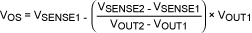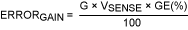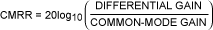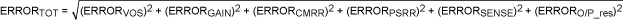# DC Error Budget Calculator Simplifies Selection of Optimal Current-Sense Amplifiers

### 要約

This article describes a systematic approach for calculating the total DC error budget of a current-sense amplifier (CSA). The individual sources of error are discussed and a method for estimating the total error budget is presented. Finally, a step-by-step process explains how to use calculator software, developed to help quickly compute the total error of the selected CSA.

A similar version of this article appeared in German in the February 6, 2013 issue of Elektronikpraxis magazine.

### Introduction

Integrated current-sense amplifiers (CSAs) are frequently used to measure the current flow in electronic circuits. They amplify the small voltage drop across a sense resistor inserted in the current path to perform critical system-level functions. Examples include overcurrent-protection and supervising devices, programmable current sources, linear and switch-mode power supplies, battery chargers, and fuel gauges. While the required current-sense specifications and implementation schemes are as diverse as these applications themselves, analysis of the CSA error budget is a fundamental component of every design. A thorough understanding of CSA error specifications and their interactions will prove immensely helpful when selecting the appropriate device for an application. Needless to say, that understanding will also minimize last-minute design iterations.
This article discusses CSA error sources and outlines a methodology for estimating the total error budget. A step-by-step process explains how to use calculator software developed by Maxim Integrated. With a simple web-based GUI, this software determines the total DC error budget for any selected Maxim CSA. An application example will familiarize the reader with the basic operation of the calculator. Design tips and message flags will alert you to any circuit conditions that are inconsistent with the CSA's specifications.

### Error Sources in a Current-Sense Amplifier

There are several sources of DC error prevalent in a CSA. Each is reviewed briefly.

### Input Offset Voltage

Similar to an op amp, the input offset voltage (VOS) of a CSA is defined as the voltage that must be applied across the CSA's inputs to drive the output voltage to zero. Offset errors are usually not measured directly, because in single-supply situations the output of the CSA cannot go below the output voltage low (VOL) limit. Hence, the input VOS is more accurately inferred as the intersection between the linear regression of the observed VOUT and the VSENSE transfer curve on the VSENSE axis as shown in Figure 1.Figure 1. A plot of output voltage versus sense voltage is used to determine the offset voltage.
If VOUT1 is the measured output voltage with VSENSE = VSENSE1, and if VOUT2 is the measured output voltage with VSENSE = VSENSE2, then VOS can be calculated with the following formula:(Eq. 1)
The output-referred offset error in the CSA that results from the input offset voltage is given as:
 ERRORVOS = G × VOS (Eq. 2)
Where G = expected gain of the amplifier.
One method to minimize the offset-voltage-error component is to choose a larger value of sense resistor. The larger value develops a higher sense voltage, thereby reducing the offset-voltage-error component from the error budget. There is a caveat, however. Selecting an external RSENSE resistor is a delicate balance between an acceptable voltage drop or power dissipation across the resistor and the offset error of the CSA. For high-current precision applications where a larger sense resistor cannot be used, a precision CSA can be a better option.

### Gain Error

Gain error is defined as the percentage deviation of the observed differential gain with respect to the ideal differential gain of the CSA. The ideal gain is either internally fixed or set by the ratio of external resistors. Gain error is specified by:(Eq. 3)
Where the observed gain is obtained from Figure 1 as:(Eq. 4)
Gain error thus measures the percent deviation of the observed slope of the transfer function with respect to the ideal slope.
The output referred error resulting from the gain error component is specified as:(Eq. 5)

### Gain Nonlinearity

A perfectly linear CSA exhibits a constant slope in the transfer curve. Usually the gain nonlinearity is negligible, compared to the offset and gain error, if the output swing is within the linear region. (This region will be specified by the Output Voltage High and Output Voltage Low specifications on the CSA's data sheet.) Hence, the error from gain nonlinearity can be safely ignored during total error budget calculations.

### Common-Mode Rejection Ratio

The common-mode rejection ratio (CMRR) measures the ability of the CSA to reject any variation in the input signals that is common to both the inputs. The CMRR specification in a data sheet is usually referred to the input. CMRR is defined by the formula:(Eq. 6)
The maximum output referred error from common-mode input voltage variation is specified as:
 ERRORCMRR = G × Maximum [Abs Value (Min VCM - Data Sheet VCM), Abs Value (Max VCM - Data Sheet VCM)] × 10-CMRR/20 (Eq. 7)
Where:
Data sheet VCM = common-mode voltage at which the CSA's gain error and offset error are characterized on the data sheet.
Min VCM = minimum common-mode voltage applied in the user's circuit.
Max VCM = maximum common-mode voltage applied in the user's circuit.

### Power-Supply Rejection Ratio

The power-supply voltage rejection ratio (PSRR) measures the ability of the CSA to reject any variation of the supply voltage, VCC. The PSRR specification on a data sheet is usually referred back to the input so that its effect can be compared with the applied differential signal. The maximum output referred error from a variation in the supply voltage is specified as:
 ERRORPSRR = G × Maximum [Abs Value (Min VDD - Data Sheet VDD), Abs Value (Max VDD - Data Sheet VDD)] × 10-PSRR/20 (Eq. 8)
Where:
Data sheet VDD = supply voltage at which the CSA's gain error and offset error are characterized on the data sheet.
Min VDD = minimum supply voltage as applied in the user's circuit
Max VDD = maximum supply voltage as applied in the user's circuit

### Sense Resistor Tolerance

Since most CSAs use an external current-sense resistor, the tolerance of the sense resistor must be also taken into account while computing the total error budget. Use tight tolerance resistors to minimize this error component. Additionally, for high-current applications, it is strongly recommended that 4-wire Kelvin connection resistors be used for best results.
The output referred error from the sense resistor's tolerance is given as:(Eq. 9)

### Output Resistor Tolerance

CSAs with current output such as the MAX9934 are often used with a termination resistor that converts the output current to a voltage. Current output has a notable advantage: multiple CSAs can be multiplexed on the same termination resistor. Another advantage of a current-output architecture is that it makes the CSA relatively immune to ground bounce errors if the output resistor is terminated to the ADC ground. Output-resistor tolerance is, however, another component that must be considered in the total error calculations. The error from the output resistor tolerance is given as:(Eq. 10)
Where GM = transconductance gain.

### System Error Budget

Designers often find it tempting to use the worst-case approach to compute the total error from the CSA. In this approach, the total error is computed as a simple summation of all the individual error sources. While the worst-case method guarantees that the error limit is never exceeded, more often than not it produces an overly conservative and inaccurate estimate. An implicit assumption made in the worst-case method is that all the individual error sources are correlated and have the same polarity.
An alternative approach is the root sum of squares (RSS) analysis. Here, the total error is the square root of the sum of squares of the individual error. RSS is based on the fact that while adding two distributions of random (normally distributed or Gaussian) measurements, the standard deviation of the resulting distribution is equal to the square root of the sum of the squares of the standard deviations of the initial distributions. The RSS method is more realistic than the worst-case method when the individual error sources are uncorrelated, as in the case of a CSA. If the guaranteed worst-case numbers of individual errors are used, then RSS analysis yields the most reasonable result.
An interesting fact about the RSS method is that, although it results in a total error that is greater than the individual error terms, the dominant error term often overshadows all the other terms.
Using the RSS method, the total error budget for a voltage-output CSA is given as:(Eq. 11)
Using the RSS method, the total error budget for a current-output CSA is given as:(Eq. 12)
It is imperative with these calculations that all the error sources are referred to the same node, usually either the input or the output. This is important because CSAs usually have a gain greater than unity, so the absolute size of the output error is bigger than the input error magnitude.

### Current-Sense Error Budget Calculator

A new calculator has been developed by Maxim Integrated to assist the designer in estimating the total error of the selected CSA. Available at no cost, the software only requires the user to input a few fields of application-specific information. It automatically prefills the relevant specifications from the selected CSA's data sheet and outputs the expected maximum total error using the RSS method. The calculator also flags the user if inadvertent mistakes are made when data is entered. For example, it will alert the user if the input sense voltage exceeds the recommended full-scale sense voltage, if the supply voltage is out of range, if the output swing constraints are not met, and so on.
To get started with the tool, click on the following link (https://www.maximintegrated.com/en/design/design-tools/calculators/general-engineering.html) and follow the instructions below:

### Using the Current-Sense Error Budget Calculator

Consider the design of an overcurrent protection circuit that requires a CSA to meet the following specifications:
1. Input trip point = 50A (unidirectional)
2. Sense-resistor tolerance = 0.5%
3. Sense resistor = < 1mΩ
4. Supply voltage range = 4.5V to 5.5V
5. Input common-mode voltage range = 12V to 18V
6. Total error budget = < 2%, which implies a CSA gain error < 2% and VOS < 1mV since the individual errors cannot exceed the total error budget

#### Step 1. Parametric search

Based on the above criteria, a parametric search shortlists the following candidate CSAs: MAX9922, MAX9918, MAX9929F, MAX4080, MAX4373, and MAX4172.

#### Step 2. Current-sense error calculator inputs

To narrow the above list based on the total error budget, use the current-sense error budget calculator. Select one of the CSAs from the Maxim CSA Device Number drop-down box and enter the application-specific input parameters (Figure 2).Figure 2. Input fields that must entered by the user.

#### Step 3. Verify the data sheet specifications

The calculator automatically fills in the maximum offset error, maximum gain error, CMRR, and PSRR specifications from the selected CSA's data sheet. By default, these specifications correspond to T = 25°C, as illustrated in Figure 3 for the MAX9922 CSA.Figure 3. Calculator prefills the relevant specifications from the selected CSA data sheet.
Now press the Calculate button to note the total error calculated by the software.

#### Step 4. Data sheet overrides

Even though the calculator prefills the gain, offset error, gain error, CMRR, and PSRR values from the data sheet, it is flexible. You can replace those values with user-specified values, if necessary. For example, the designer might have a provision to calibrate and remove the effect of offset voltage in software, in which case a nonprecision CSA may also meet the error budget requirements. Another user may be more interested in worst-case temperature specs from a data sheet instead of the default T = 25°C.
To substitute values for the prefilled numbers, use the Enter Overrides field to make application-specific adjustments. Returning to the MAX9922 example, press the Calculate button resulted in the error shown in Figure 4. The calculator prompts the user to reduce the gain because the output voltage cannot exceed the Output Voltage High limit of the part.
Since the MAX9922's gain is adjustable, simply reduce the gain to 60V/V in the corresponding Data sheet Overrides field. After updating the gain, Figure 5 shows the resulting total error budget.

#### Step 5. Selecting a different CSA

To evaluate the error budget of the next CSA under consideration, e.g., the MAX9918, simply change the selection in the Maxim CSA Device Number drop-down box. There is no need to reenter the input parameters. Click the Calculate button after each CSA selection to get the corresponding error budget. Table 1 lists the error budget numbers for all the CSAs shortlisted for this example. Results show that only the MAX9922 and MAX9918 meet the total error budget criteria of this application.
 Table 1. Total Error Budget Computed Using the Calculator Part Total Error (%) Conditions T = 25°C T = -40°C to +85°C MAX9922 0.64 0.79 Gain = 60 MAX9918 1.13 1.93 Gain = 60 MAX9929F 1.39 3.45 — MAX4080S 1.43 2.55 — MAX4373F 2.84 6.18 — MAX4172 — 2.57 O/P termination = 6kΩ

### Summary

This article introduces the Current-Sense Error Budget Calculator, a powerful tool for identifying CSA choices in a fast and logical manner. The article also explains the importance of developing a better understanding of error specifications for CSAs. Together, this knowledge and the calculator will assist the designer in selecting the optimal CSA for an application. The RSS method of error analysis that is the foundation of the calculator can be further extended to compute system-level accuracy performance for several electronic components or circuits.